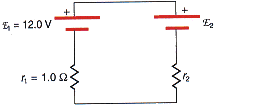# Terminal voltage and current flow

## Homework Statement(a) Calculate the magnitude and indicate the direction of flow of current in Figure 20.40. E2 = 19.0 V and r2 = 0.55 Ω.

(b) Find the terminal voltage of each battery.

V = Ir

## The Attempt at a Solution

I found the answer for part a to be 4.52 but i don't know how to get the second one.

berkeman
Mentor

## Homework Statement(a) Calculate the magnitude and indicate the direction of flow of current in Figure 20.40. E2 = 19.0 V and r2 = 0.55 Ω.

(b) Find the terminal voltage of each battery.

V = Ir

## The Attempt at a Solution

I found the answer for part a to be 4.52 but i don't know how to get the second one.

I don't understand the question. It looks like you are given the battery voltages...